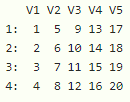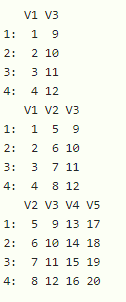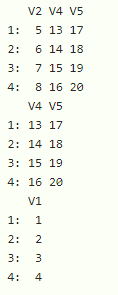GFG App
Open AppBrowser
Continue

# Select Subset of DataTable Columns in R

In this article, we will discuss how to select a subset of data table columns in R programming language.

Let’s create a data table using a matrix. First, we need to load data.table package in the working space.

Installation

install.packages(“data.table”)

library(“data.table”)

Dataset in use:## Method 1: Using []

We can select a subset of datatable columns by index operator – []

Syntax:

datatable[ ,  c(columns), with = FALSE]

Where,

• datatable is the input data table
• columns are the columns in the datatable to be selected
• with =FALSE is an optional parameter

Example: R program to select subset of columns from the data table

## R

 `# load data.table package ` `library``(``"data.table"``) ` ` `  `# create data table with matrix with 20 elements ` `# 4 rows and 5 columns ` `data= ``data.table``(``matrix``(1:20, nrow=4,ncol = 5))              ` ` `  `# display the subset that include v1 and v3 columns ` `print``(data[ ,  ``c``(``"V1"``, ``"V3"``), with = ``FALSE``]) ` ` `  `# display the subset that include v1 , v2 and v3 columns ` `print``(data[ ,  ``c``(``"V1"``,``"V2"``, ``"V3"``), with = ``FALSE``]) ` ` `  `# display the subset that include v2,v3,v4 and v5 columns ` `print``(data[ ,  ``c``(``"V2"``, ``"V3"``,``"V4"``,``"V5"``), with = ``FALSE``])`

Output:## Method 2: Using !

Using ! operator before columns can be enough to get the job done by this approach. Here we are not including the subset that is selected from the data table

Syntax:

datatable[ ,  !c(columns), with = FALSE]

where,

• datatable is the input data table
• columns are the columns in the datatable to be selected

Example: R program to select columns from datatable

## R

 `# load data.table package ` `library``(``"data.table"``) ` ` `  `# create data table with matrix with 20 elements ` `# 4 rows and 5 columns ` `data= ``data.table``(``matrix``(1:20, nrow=4,ncol = 5))              ` ` `  `# display the subset that exclude v1 and v3 columns ` `print``(data[ ,  !``c``(``"V1"``, ``"V3"``), with = ``FALSE``]) ` ` `  `# display the subset that exclude  v1 , v2 and v3 columns ` `print``(data[ ,  !``c``(``"V1"``,``"V2"``, ``"V3"``), with = ``FALSE``]) ` ` `  `# display the subset that exclude v2,v3,v4 and v5 columns ` `print``(data[ ,  !``c``(``"V2"``, ``"V3"``,``"V4"``,``"V5"``), with = ``FALSE``])`

Output:My Personal Notes arrow_drop_up